### Home > A2C > Chapter 4 > Lesson 4.2.4 > Problem4-125

4-125.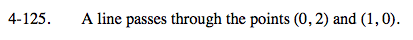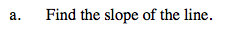Plot the points on a graph. Find the slope (rise over run).

$\textit{m}=\frac{\Delta\textit{y}}{\Delta\textit{x}}=\frac{2-0}{0-1}=-2$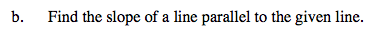Remember, parallel lines have the same slope.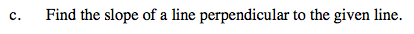Remember, perpendicular lines form a right angle.
The slopes will be the negative reciprocals of each other.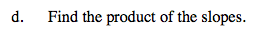Use the values from parts (b) and (c).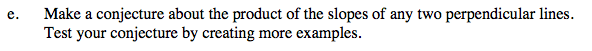The product of the slopes of two perpendicular lines is always the same number.

Use the eTool below to explore the line.
Click the link at right for the full version of the eTool: A2C 4-125 HW eTool